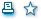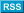HomeResearchResearchDepartment of Applied Mathematics and Modeling Български
 Faculty of Mathematics and Informatics - Department of Applied Mathematics and ModelingDepartment of Applied Mathematics and Modeling Research directions     Iterational methods for simultaneous finding of zeros of polynomial equations Qualitative methods of impulsive equations Impulsive models in population dynamics. Models with random impulses Stability of the solutions of differential equations Analytical, statistical and numerical modeling of gas lasers Modeling of gas discharge and breakdown curves Mathematical and statistical modeling in food technologies Spherical codes and designs Stability and convergence analysis of difference schemes for approximate solutions of partial differential equations. Analysis of non-linear multidimensional problems Numerical modelling of fluid dynamics problems for drainage and rupture of a free thin film under the action of different forces Statistical treatment of information                                           Chair: Prof. Khristo Semerdzhiev, DSc
 More...